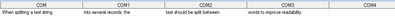## Macro variable sequence in data step

I created a data step macro to split a long string into a fixed length but also maintain text readability by splitting at the space closest to the fixed length. For example, a comment has 113 characters. To split it into 4 pieces each with max of 30 characters. The macro works, but I don't know how to simplify &Var.1, &Var.2 etc. There should be a simple way to say like else &var.i=.... Below is the macro and the test.

```%macro split_text(txt=,var=);
length __txt \$2000;
__txt=&txt;
m=30;
len=length(__txt);
n=floor(len/m);

do i=0 to n;
j=find(__txt,' ',-m);
if i=0 then &var=substr(__txt,1,j-1);
if i=1 then &var.1=substr(__txt,1,j-1);
if i=2 then &var.2=substr(__txt,1,j-1);
if i=3 then &var.3=substr(__txt,1,j-1);
if i=4 then &var.4=substr(__txt,1,j-1);

*reset;
__txt=substr(__txt,j+1);
end;
%mend;

data test;
comment = 'When splitting a text string into several records, the text should be split between words to improve readability.';
%split_text(txt=comment,var=COM);
run;```

Below is the result.1 ACCEPTED SOLUTION

Accepted Solutions

## Re: Macro variable sequence in data step

Maybe what is missing is an array. Something like this might help (untested):

``````%macro split_text(txt=,var=);
length __txt \$2000;
__txt=&txt;
m=30;
len=length(__txt);
n=floor(len/m);
array vars (*) \$ 30 &var.0 - &var.4;
do i=0 to n;
j=find(__txt,' ',-m);
vars(i) = substr(__txt,1,j-1);
*reset;
__txt=substr(__txt,j+1);
end;
%mend;``````
6 REPLIES 6

## Re: Macro variable sequence in data step

Maybe what is missing is an array. Something like this might help (untested):

``````%macro split_text(txt=,var=);
length __txt \$2000;
__txt=&txt;
m=30;
len=length(__txt);
n=floor(len/m);
array vars (*) \$ 30 &var.0 - &var.4;
do i=0 to n;
j=find(__txt,' ',-m);
vars(i) = substr(__txt,1,j-1);
*reset;
__txt=substr(__txt,j+1);
end;
%mend;``````

## Re: Macro variable sequence in data step

Thanks a lot! It works!

Another question: how to dynamically assign exact number of variables based on string length? Extra variables with blank values should be removed.

## Re: Macro variable sequence in data step

What would the rule be, based on string length? Would it be find the longest string in your dataset and divide by 30? Lets say your maximum string length was 1024 (based on the position of the rightmost character in the string). 1024 / 30 is 34.13. That means you will need 35 30-character variables to store this. You can easily calculate it like this and put the result into macro variable you can use in following code:

``````proc sql;
select ceil(max(length(string))/30)
into :VarNum
from MyLib.MyTable;
quit;
%put VarNum = &VarNum;
``````

## Re: Macro variable sequence in data step

Sorry, I did not make myself clear.

I mean in data step, assigning array with &var.1-&var.4 is based on current string length of 113. But if next string length is only 70, then only &var.1-&var.3 is needed. How to assign array with exact number of variables based on the string length?

## Re: Macro variable sequence in data step

You solve this by splitting vertically instead of horizontally. You will have a dataset with three variables (a key for identification, a sequence number, and the text) with a variable number of observations per key. This is the most efficient way to store variable-length texts in smaller variables

## Re: Macro variable sequence in data step

Thanks Kurt!

I raised the 2nd question just want to be perfect.

In reality I can handle it with one more step "if not blank, then output to dataset".

Discussion stats
• 6 replies
• 301 views
• 0 likes
• 3 in conversation